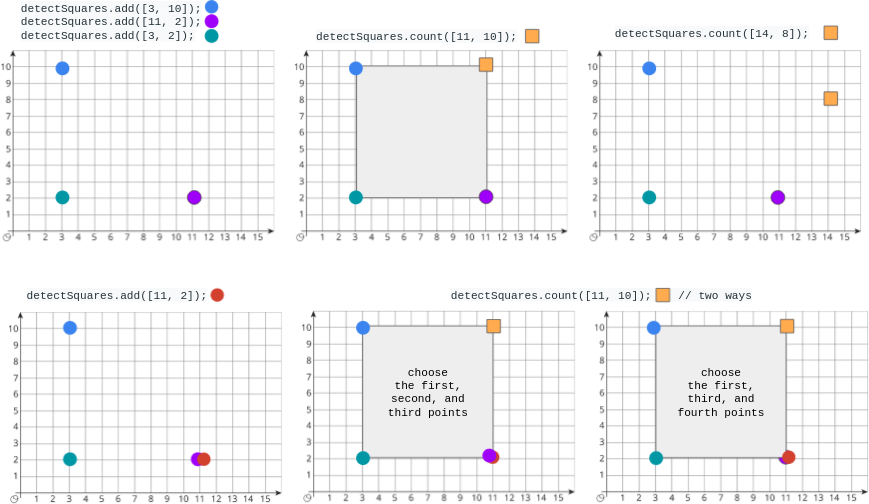# 2013 Detect Squares

You are given a stream of points on the X-Y plane. Design an algorithm that:

• Adds new points from the stream into a data structure. Duplicate points are allowed and should be treated as different points.
• Given a query point, counts the number of ways to choose three points from the data structure such that the three points and the query point form an axis-aligned square with positive area.

An axis-aligned square is a square whose edges are all the same length and are either parallel or perpendicular to the x-axis and y-axis.

Implement the `DetectSquares` class:

• `DetectSquares()` Initializes the object with an empty data structure.
• `void add(int[] point)` Adds a new point `point = [x, y]` to the data structure.
• `int count(int[] point)` Counts the number of ways to form axis-aligned squares with point `point = [x, y]` as described above.

Example 1:``````Input
[[], [[3, 10]], [[11, 2]], [[3, 2]], [[11, 10]], [[14, 8]], [[11, 2]], [[11, 10]]]
Output
[null, null, null, null, 1, 0, null, 2]

Explanation
DetectSquares detectSquares = new DetectSquares();
detectSquares.count([11, 10]); // return 1. You can choose:
//   - The first, second, and third points
detectSquares.count([14, 8]);  // return 0. The query point cannot form a square
// with any points in the data structure.
• `point.length == 2`
• `0 <= x, y <= 1000`
• At most `3000` calls in total will be made to `add` and `count`.
 `````` 1 2 3 4 5 6 7 8 9 10 11 12 13 14 15 16 17 18 19 20 21 22 23 24 `````` ``````class DetectSquares: def __init__(self): self.points = defaultdict(int) # (x,y): count def add(self, point: List[int]) -> None: self.points[tuple(point)] += 1 def count(self, point: List[int]) -> int: res = 0 x1, y1 = point for (x2, y2), n in self.points.items(): dx, dy = abs(x1-x2), abs(y1-y2) if dx == dy and dx > 0: # point at diagonal, c1, c2 are points at anti-diagonal c1, c2 = (x1, y2), (x2, y1) if c1 in self.points and c2 in self.points: res += n * self.points[c1] * self.points[c2] return res # Your DetectSquares object will be instantiated and called as such: # obj = DetectSquares() # obj.add(point) # param_2 = obj.count(point)``````